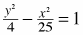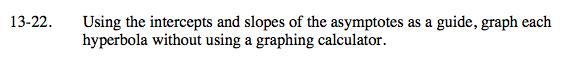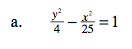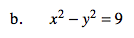### Home > A2C > Chapter 13 > Lesson 13.1.1 > Problem13-22

13-22.
1. Using the intercepts and slopes of the asymptotes as a guide, graph each hyperbola without using a graphing calculator. Homework Help ✎

1.2. x2y2 = 9$\text{Intercepts: } (0,\pm2)$

$\text{Asymptotes: } y=\pm\frac{2}{5}x$Intercepts: (±3, 0)
Asymptotes: y = ± x